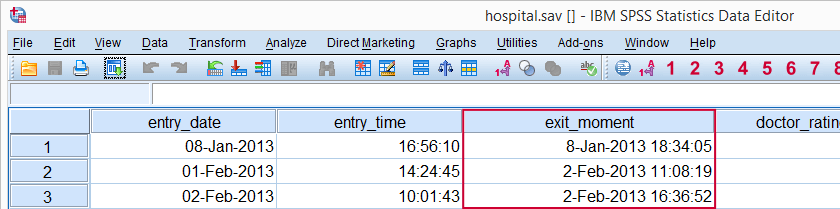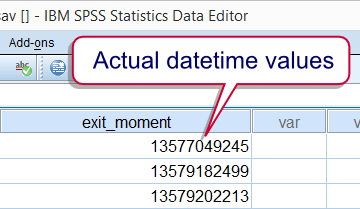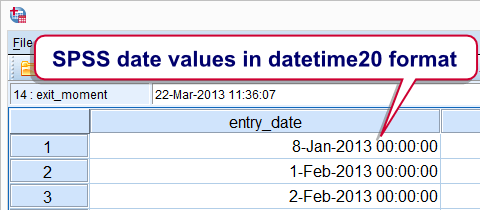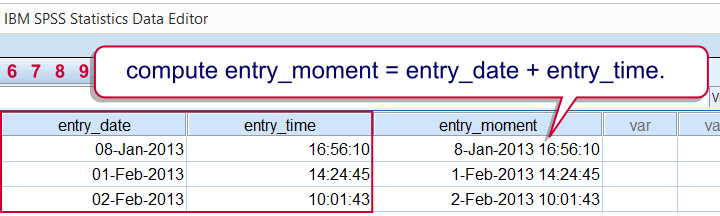SPSS TUTORIALS BASICS ANOVA REGRESSION FACTOR CORRELATION

# SPSS Datetime Variables Basics

Working with SPSS datetime variables is not hard at all if you understand some basics. This tutorial walks you through just those. Those who'd like to follow along may download and open hospital.sav.## SPSS Datetime Variables - What Are They?

SPSS datetime variables are variables that hold the numbers of seconds between the year 1582 and a given time on a given date. SPSS datetime values may look complicated (containing letters of months and dashes) but their values are really nothing more than huge numbers. The actual values are shown by specifying an f format, the syntax for which is formats exit_moment(f1).Note that this doesn't change the values in any way; they're merely displayed differently. Don't let their unusual appearance fool you: SPSS datetime variables are numeric variables. This implies that all standard numeric functions can be used on them. However, for calculations on SPSS datetime variables we'll mostly use SPSS date functions.

## SPSS Datetime Formats

We just saw that SPSS datetime values really are huge numbers of seconds. For displaying them as normal dates with times, set their format to one of the two formats outlined below.

Variable TypeFormat familyFormat (example)Shown as
NumericDatetimeDatetime178-Jan-2013 18:34
NumericDatetimeDatetime208-Jan-2013 18:34:05

## Date, Time and Datetime

The relation between SPSS date variables, time variables and datetime variables can be seen from a quick comparison of their definitions:

• SPSS date variables contain the number of seconds between 1582 and the very start (midnight) of a given date;
• SPSS time variables contain the number of seconds between the very start (midnight) of a date and some given time;
• SPSS datetime variables contain the number of seconds between 1582 and a given time on a given date.

We conclude from this that SPSS date values can be seen as datetime values with a 00:00:00 time component. Running formats entry_date(datetime20). confirms this; SPSS date values can be properly displayed as datetime20 because their actual values are very similar to datetime values.Reversely, datetime values can be displayed as dates as well. If doing so, just keep in mind that the time component does not disappear by no longer displaying it.

## SPSS Datetime from Date and Time

At this point we may see that SPSS datetime values are simply the sum of a date value and a time value. Running the syntax below confirms this.

*1. Date (seconds) + time (seconds) = datetime (seconds).

compute entry_moment = entry_date + entry_time.
exe.

*2. Show seconds as normal date with time.

formats entry_moment(datetime20).Combining date and time into datetime - it really that simple.

## SPSS Extract Date from Datetime

SPSS users who understand datetime variables will rarely -if ever- want to extract their date components. For the sake of completeness, the official way is to create the date variable using DATE.DMY. We obtain the required day, month and year components by applying XDATE to the datetime variable.
Note the day of the month is captured by XDATE.MDAY; XDATE.DAY is not valid in SPSS.In the syntax below, we first create day, month and year as intermediate variables before combining them with DATE.DMY. This step may be skipped by substituting XDATE into DATE.DMY which we'll demonstrate when extracting time values from datetime values.

*1. Extract date, month, and year into new variables.

compute day = xdate.mday(exit_moment).
compute month = xdate.month(exit_moment).
compute year = xdate.year(exit_moment).
exe.

*2. Compute date from (extracted) day, month and year components.

compute exit_date = date.dmy(day,month,year).
exe.

*3. Display as normal date values.

formats exit_date(date11).

The unofficial way to extract date values from datetime values uses SPSS TRUNC function; we remove the time portion from datetime values by rounding them down to 86400 seconds (one day).

*1. Delete exit_date before recomputing it.

delete variables exit_date.

*2. Extract date from datetime by TRUNC function.

compute exit_date = trunc(exit_moment,86400).
exe.

*3. Show date in date format.

formats exit_date(date11).

## SPSS Extract Time from Datetime

SPSS time values can be created from hours, minutes and seconds by TIME.HMS. Again, these components can be extracted from datetime values by using XDATE as shown in the syntax below.

*1. Compute time values by combining hour, minute, second components extracted from datetime values.

compute exit_time = time.hms(xdate.hour(exit_moment),xdate.minute(exit_moment),xdate.second(exit_moment)).
exe.

*2. Show seconds as normal times.

formats exit_time(time8).

A faster alternative here is using SPSS MOD function; we basically throw away the date component by removing all 86400-folds (a day has 86400 seconds) from the datetime values.

*1. Remove date component from datetime values.

compute exit_time = mod(exit_moment,86400).
exe.

*2. Show seconds as normal times.

formats exit_time(time8).

# Tell us what you think!

*Required field. Your comment will show up after approval from a moderator.

# THIS TUTORIAL HAS 3 COMMENTS:

•### By ALBERTO ZUCCHI on April 12th, 2016

Thanks for tutorial! I'd like to know how to define the weekday on the basis of calendar date (eg, 01/01/2015 was Monday, 01/02/2015 was Sunday, an so on). Many thanks for help!

•### By Ruben Geert van den Berg on April 12th, 2016

Hi Alberto!

Great question!! I wrote a tiny syntax example on it: SPSS Extract Weekdays from Date Variable.

Hope that helps!

•### By ALBERTO ZUCCHI on April 13th, 2016

Thanks, Ruben, it was very helpful! I've found that applying exactly your syntax there was a little problem, i.e. there was a delay of one calendar day. So I modified the syntax this way:

```compute mydate = datesum((date.dmy(1,1,2015)-1),\$casenum,'days'). formats mydate(date11). execute.```

That is, I simply detracted -1 day; so it worked perfect. Many thanks again, my best regards!